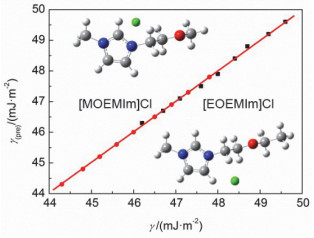%A Yanyan GONG,Haichun LIU,Duo ZHANG,Jing TONG %T Thermodynamic Properties of the Ether-Based Functionalized Ionic Liquids [MOEMIm]Cl and [EOEMIm]Cl %0 Journal Article %D 2019 %J Acta Phys. -Chim. Sin. %R 10.3866/PKU.WHXB201901072 %P 1224-1231 %V 35 %N 11 %U {https://www.whxb.pku.edu.cn/CN/abstract/article_34990.shtml} %8 2019-09-02 %X

Functionalized ionic liquids containing ether groups can be obtained through reasonable design. The unique properties of ether-based functionalized ionic liquids are attractive for a variety of practical applications. To further develop these ether-based functionalized ionic liquids, it is important to accurately describe the thermophysical properties of ether-based functionalized ionic liquids and to predict, simulate, and optimize theoretical models of thermophysical properties. Herein, the ether-functionalized ionic liquids [MOEMIm]Cl and [EOEMIm]Cl were characterized by nuclear magnetic resonance spectroscopy, elemental analysis, and thermogravimetric analysis. The density (ρ), surface tension (γ), and refractive index (nD) of [MOEMIm]Cl and [EOEMIm]Cl were measured at 5 K intervals between 288.15 and 328.15 K. Based on the obtained experimental data, the molar volume (V), molecular volume (Vm), standard entropy (S(298)0), and lattice energy (UPOT) of [MOEMIm]Cl and [EOEMIm]Cl were calculated and the data obtained at 298.15 K were compared. The comparison results agreed well with the literature values within the experimental error range and indicated that both [MOEMIm]Cl and [EOEMIm]Cl exhibit small lattice energies and are in the molten state at room temperature (T = 298.15 K). Based on these experimental data, the molar surface Gibbs free energy (gs), molar surface entropy (s), molar surface enthalpy (h), molar polarization (Rm), and molar polarizability (αp) of [MOEMIm]Cl and [EOEMIm]Cl were also calculated. The calculation results show that the molar surface enthalpy (h) is approximately constant, that is, the molar surface constant pressure heat capacity is close to zero, indicating that the process of heat capacity change from the inside to the surface of the ionic liquid is an equivalent coulomb process. Simultaneously, the calculation results showed that the molar polarization (Rm) and molar polarizability (αp) of the ionic liquids were independent of temperature, indicating that Rm and αp reflect the induced dipole effect of the ionic liquid. The molar surface Gibbs free energy definition equation was combined with the Lorentz-Lorenz equation to obtain a novel modified Lorentz-Lorenz equation and was used to predict the surface tension of the [MOEMIm]Cl and [EOEMIm]Cl liquids. The values predicted using this combined equation were highly correlated with the experimental values.